# 数据结构笔记（六）： 图的存储与遍历

## 演示

• 基本图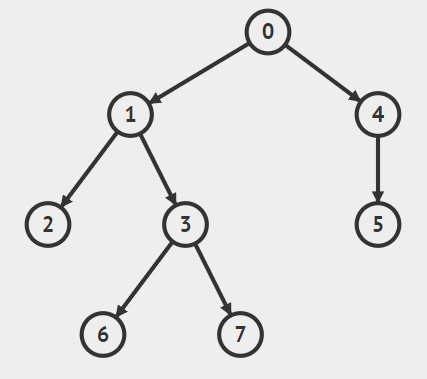• 深度遍历(从0开始)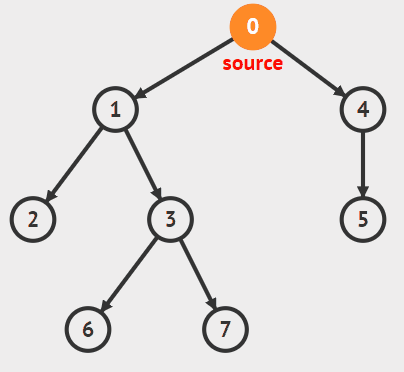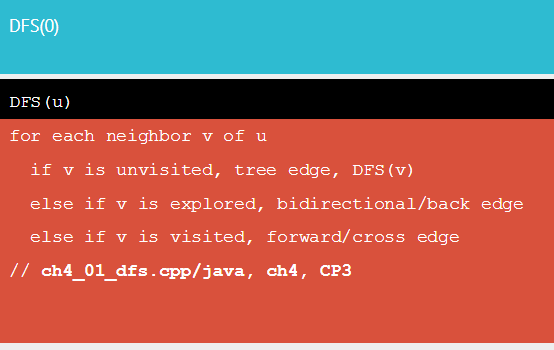• 广度遍历（从0开始）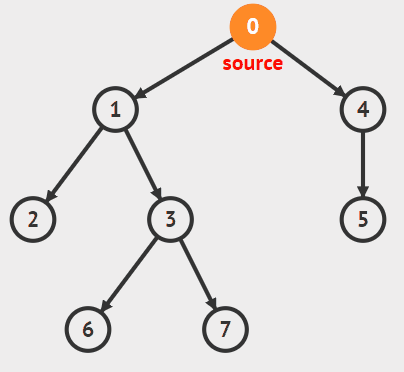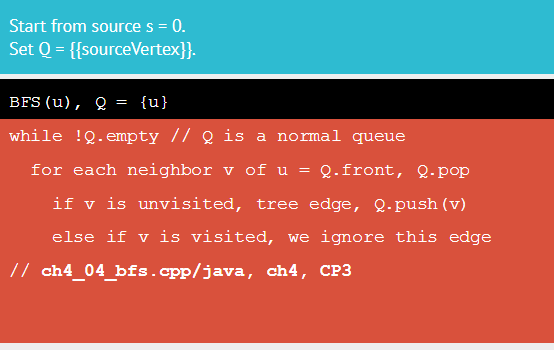## 代码

### 邻接矩阵存储

``````#include <iostream>
#include <cstdio>
#include <cstring>
#include <vector>
#include <queue>
#include <algorithm>
using namespace std;
const int maxn = 1010;
int G[maxn][maxn]; // 邻接矩阵
bool vis1[maxn]; // dfs标记数组
bool vis2[maxn]; // bfs标记数组
int n, m; // 点数与边数

void init() // 初始化
{
memset(G, 0, sizeof(G)); // 为0表示无边相连
memset(vis1, 0, sizeof(vis1)); // 为0表示未被访问
memset(vis2, 0, sizeof(vis2));
}

void dfs(int u) // 深度优先遍历
{
cout << u << " ";
vis1[u] = true;
for (int i = 1; i <= n; ++i)
{
if (!vis1[i] && G[u][i]) // 没有被访问过且有边
dfs(i);
}
}

void bfs(int u) // 广度优先遍历
{
int now = u;
vis2[now] = true;
queue<int>Q;
Q.push(now);
while (!Q.empty())
{
now = Q.front();
Q.pop();
cout << now << " ";
for (int i = 1; i <= n; ++i)
{
if (!vis2[i] && G[now][i]) // 没有被访问过且有边
{
Q.push(i);
vis2[i] = true;
}
}
}
}

int main()
{
while (cin >> n >> m)
{
init();
int x, y;
for (int i = 1; i <= m; ++i)
{
cin >> x >> y;
G[x][y] = G[y][x] = 1; // 无向边
}
cout << "深度优先遍历：" << endl;
for (int i = 1; i <= n; ++i)
{
if (!vis1[i])
dfs(i);
}
cout << endl;
cout << "广度优先遍历：" << endl;
for (int i = 1; i <= n; ++i)
{
if (!vis2[i])
bfs(i);
}
cout << endl;
}
return 0;
}

/*
9 7
1 2
1 4
2 6
2 5
4 7
3 8
3 9

1 2 5 6 4 7 3 8 9

1 2 4 5 6 7 3 8 9
*/
``````

### 邻接表存储（链表）

``````#include <iostream>
#include <cstdio>
#include <cstring>
#include <vector>
#include <queue>
#include <algorithm>
using namespace std;
const int maxn = 1010;
bool vis1[maxn]; // dfs标记数组
bool vis2[maxn]; // bfs标记数组
int n, m; // 点数与边数

struct Edge
{
int to;
Edge * next;
Edge(int a = 0, Edge * b = NULL):to(a), next(b){} // 默认构造函数
};

Edge * Vertex[maxn] = {NULL}; // 初始化为空

void addedge(int x, int y) // “连边”
{
Edge * pt = new Edge(y, Vertex[x]); // 总是从第一个插入
Vertex[x] = pt;
}

void init() // 初始化
{
memset(vis1, 0, sizeof(vis1)); // 为0表示未被访问
memset(vis2, 0, sizeof(vis2));
}

void dfs(int u) // 深度优先遍历
{
cout << u << " ";
vis1[u] = true;
for (Edge * pt = Vertex[u]; pt; pt = pt->next)
{
if (!vis1[pt->to])
dfs(pt->to);
}
}

void bfs(int u) // 广度优先遍历
{
int now = u;
vis2[now] = true;
queue<int> Q;
Q.push(now);
while (!Q.empty())
{
now = Q.front();
Q.pop();
cout << now << " ";
for (Edge * pt = Vertex[now]; pt; pt = pt->next)
{
if (!vis2[pt->to])
{
Q.push(pt->to);
vis2[pt->to] = true;
}
}
}
}

int main()
{
while (cin >> n >> m)
{
init();
int a, b;
for (int i = 1; i <= m; ++i)
{
cin >> a >> b;
}
cout << "深度优先遍历：" << endl;
for (int i = 1; i <= n; ++i)
{
if (!vis1[i])
dfs(i);
}
cout << endl;
cout << "广度优先遍历：" << endl;
for (int i = 1; i <= n; ++i)
{
if (!vis2[i])
bfs(i);
}
cout << endl;
}
return 0;
}

/*
9 7
1 2
1 4
2 6
2 5
4 7
3 8
3 9

1 4 7 2 5 6 3 9 8

1 4 2 7 5 6 3 9 8
*/
``````

### 邻接表存储（顺序表）

``````#include <iostream>
#include <cstdio>
#include <cstring>
#include <vector>
#include <queue>
#include <algorithm>
using namespace std;
const int maxn = 1010;
bool vis1[maxn]; // dfs标记数组
bool vis2[maxn]; // bfs标记数组
int n, m; // 点数与边数

vector <int> G[maxn];

void init() // 初始化
{
memset(vis1, 0, sizeof(vis1)); // 为0表示未被访问
memset(vis2, 0, sizeof(vis2));
}

void dfs(int u) // 深度优先遍历
{
cout << u << " ";
vis1[u] = true;
for (int i = 0; i < G[u].size(); ++i)
{
if (!vis1[G[u][i]])
dfs(G[u][i]);
}
}

void bfs(int u) // 广度优先遍历
{
int now = u;
vis2[now] = true;
queue<int> Q;
Q.push(now);
while (!Q.empty())
{
now = Q.front();
Q.pop();
cout << now << " ";
for (int i = 0; i < G[now].size(); ++i)
{
if (!vis2[G[now][i]])
{
Q.push(G[now][i]);
vis2[G[now][i]] = true;
}
}
}
}

int main()
{
while (cin >> n >> m)
{
init();
int x, y;
for (int i = 1; i <= m; ++i)
{
cin >> x >> y;
G[x].push_back(y);
G[y].push_back(x);
}

// 按照大小顺序依次输出
//for(int i=1;i<=n;++i)
//    sort(G[i].begin(),G[i].end());

cout << "深度优先遍历：" << endl;
for (int i = 1; i <= n; ++i)
{
if (!vis1[i])
dfs(i);
}
cout << endl;
cout << "广度优先遍历：" << endl;
for (int i = 1; i <= n; ++i)
{
if (!vis2[i])
bfs(i);
}
cout << endl;
}
return 0;
}

/*
9 7
1 2
1 4
2 6
2 5
4 7
3 8
3 9

1 2 6 5 4 7 3 8 9

1 2 4 6 5 7 3 8 9
*/
``````

## 基础知识

### 邻接矩阵### 邻接表The end.
2017-11-11 星期六

Not Comment Found